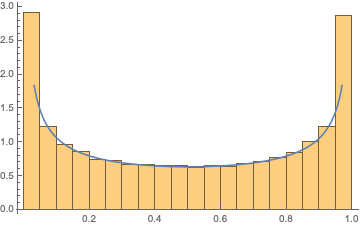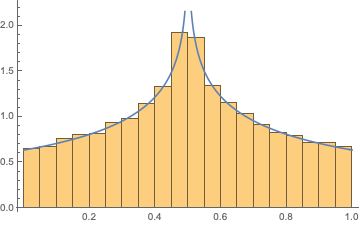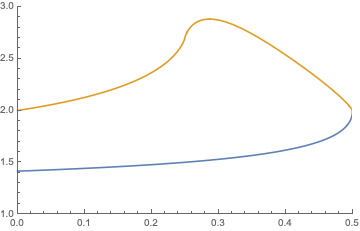Random matrix with given singular values

Let $$\sigma_1\geq\sigma_2\geq...\geq\sigma_n\geq0$$ be any deterministic sequence of positive real numbers such that $$\sum_{i=1}^n\sigma_i^2=1$$. Let $$D=diag\{\sigma_1,...,\sigma_n\}\in\mathbb{R}^{n\times n}$$ be a diagonal matrix of size $$n\times n$$.

Let $$U$$ and $$V$$ be two independent random matrices uniformly distributed on the orthogonal group $$O(n)$$. Then we form a random matrix as follows: $$A=UDV^T$$ Therefore, $$A$$ is a random matrix with given singular values and the distribution is orthogonally invariant. Now we define a quantity that measures how far away is $$A$$ from a diagonal matrix: $$f_{\sigma}(A)=\sum_{1\leq i\neq j\leq n}A_{ij}^2=\sum_{1\leq i\neq j\leq n}\left(\sum_{k=1}^n\sigma_kU_{ik}V_{jk}\right)^2=1-\sum_{i=1}^n\left(\sum_{j=1}^n\sigma_jU_{ij}V_{ij}\right)^2$$ which is just the sum of squares of all off-diagonal elements of $$A$$, the smaller $$f_{\sigma}(A)$$ is, the "more diagonal" $$A$$ is. Note the dependency of $$f$$ on the given singular values $$\sigma$$.

I'm interested in the following quantity: $$g_{\sigma}(t)=\frac{\mathbb{P}\left(f_{\sigma}(A)\leq 2t\right)}{\mathbb{P}\left(f_{\sigma}(A)\leq t\right)}$$ where $$0.

I have the following 2 conjectures:

1. For $$n\geq 5$$, for any $$0, $$g_{\sigma}(t)$$ is maximized when $$\sigma_1=...=\sigma_n=1/\sqrt{n}$$.
2. There exists a constant $$C>1$$ independent of $$t, \sigma$$, such that $$g_{\sigma}(t)\leq C^{n^2}$$

I believe they should be correct but I have no clue of how to prove them. Any suggestions and discussions are appreciated. What kind of tools could possibly be useful?

I have a feeling that existing literature in random matrix mainly focus on going from the matrix to eigenvalues or singular values, ignoring the eigenvectors or singular vectors. I do not see results about going from given spectrum to the matrix.

• Is uniform distribution on $O(n)$ defined by the euclidean measure on spheres ? Jan 7 '20 at 18:43
• @ClaudeChaunier It is the Haar measure on the orthogonal group. Jan 7 '20 at 19:46

Conjecture 1 is false. Here is the counterexample for $$n=2$$.
this is the conjecture 1 as originally given by the OP; I see that it has now been changed.

I take $$n=2$$, set $$\sigma_1=\cos\alpha$$, $$\sigma_2=\sin\alpha$$, with $$0\leq\alpha\leq\pi/4$$, and parameterize the orthogonal matrices as $$U=\begin{pmatrix} \cos\phi&\sin\phi\\ -\sin\phi&\cos\phi \end{pmatrix},\;\;V=\begin{pmatrix} \cos\phi'&\sin\phi'\\ -\sin\phi'&\cos\phi' \end{pmatrix}.$$ The Haar measure on $$\text{SO}(2)$$ is a uniform distribution of the angles $$\phi,\phi'\in(0,2\pi)$$, with $$\phi$$ independent of $$\phi'$$. I calculate $$A=U\,\text{diag}\,(\sigma_1,\sigma_2)V^T$$ and evaluate $$f_\alpha=A_{12}^2+A_{21}^2=\tfrac{1}{2} (1-\sin 2 \alpha \sin 2\phi \sin 2\phi'-\cos 2\phi \cos 2\phi').$$

Let me now compare the two extreme cases $$\alpha=\pi/4$$ and $$\alpha=0$$, $$f_{\pi/4}=\sin^2(\phi-\phi'),\;\;f_0=\tfrac{1}{2}(1-\cos 2\phi\cos 2\phi').$$ The corresponding probability distributions are $$p_{\pi/4}(f)=\frac{1}{\pi}f^{-1/2}(1-f)^{-1/2},$$ $$p_0(f)=\frac{4}{\pi^2} \int_0^{\arccos|1-2f|}\frac{d\phi}{\sqrt{\cos^2 \phi-(1-2f)^2}}.$$ (The expression for $$p_0(f)$$ is an elliptic integral.) I checked both distributions numerically (by generating random $$\phi,\phi'$$) and they do seem to be correct, see the histograms:Because $$p_{\pi/4}(f)$$ has a peak at $$f=0$$, while $$p_0(f)$$ has a peak at $$f=1/2$$, the ratio $$g_\alpha(t)$$ of cumulative distributions at $$2t$$ and $$t$$ is larger for $$\alpha=0$$ than it is for $$\alpha=\pi/4$$, for all $$0. Here is a plot that compares the two, blue is for $$\alpha=\pi/4$$ and gold is for $$\alpha=0$$.So this is a counter example to conjecture 1, because $$\alpha=\pi/4$$ corresponds to $$\sigma_1=\sigma_2=1/\sqrt n$$ for $$n=2$$.

• I got a somewhat different expression for $f$... However, from a geometric perspective, if $\alpha=0$, the dimension of the problem is essentially reduced, the enlargement of the sublevel set of $f$ on the orthogonal group should be smaller. Jan 7 '20 at 19:45
• This is very counter intuitive and it is surprising that $\alpha=0$ consistently beats $\alpha=\pi/4$. Could the conjecture be the other way around, which means the most unbalanced case, $\sigma_1=1$ will dominate all other $\sigma$? Jan 8 '20 at 2:01
• here is my intuition: If I partition an orthogonal matrix $M$ into four blocks, $M_{11}$, $M_{12}$, $M_{21}$, and $M_{22}$, and consider the singular values of an off-diagonal block, then the distribution of these singular values peaks at 0 and at 1 when $M$ is uniformly distributed in ${\rm O}(n)$. Now if you choose the balanced case, then $M=UV^T/\sqrt n$ has a uniform distribution in $\text{O}(n)$. This means that $M$ is either largely off-diagonal (peak at 0) or largely diagonal (peak at 1), with equal probability. The peak at 0 skewes your $g$-ratio to small values. Jan 8 '20 at 7:33
• I also did some simulation. However, for $n>2$, the peak at $0$ no longer exists. Also, as $n$ gets larger, the distribution of $f$ for the two extreme cases gets closer and closer. Jan 8 '20 at 21:36
• Also, my simulation result shows that as $n$ even larger, say, > 5, the distribution of balanced case is more concentrated while the unbalanced case is slightly more spread out. Jan 8 '20 at 21:46

What you want for 2- seems to me similar to a large deviation result (https://en.wikipedia.org/wiki/Large_deviations_theory) of speed $$n^2$$ for the diagonal term $$\frac{1}{n^2}\log\mathbb{P}(\sum_i A_{ii}^2\geq s)\approx I(s)$$

with some function $$I(s)<0$$. Indeed if so we have $$\log (g(t)) \approx n^2 (I(1-2t)-I(1-t))$$ and then you can choose $$\log C=\inf (I(1-2t)-I(1-t))$$

This is not true for the case $$\sigma=(1,0,\cdots,0)$$ but we have a better upper bound. Here for $$A_{11}^2\geq s$$ it is enough to ask that $$U_{11}\geq s^{1/4}$$ and $$V_{11}\geq s^{1/4}$$. The first column of $$U$$ is a random vector chosen uniformly on the sphere $$\mathbb{S}^{n-1}$$ and a good way to construct it is using a set of iid Gaussian variables $$Y_i$$ and write $$U_{i1}:=\frac{Y_i}{\sqrt{\sum_{j}Y_j^2}}$$ We use here that the direction of a Gaussian vector is isotropic on $$\mathbb{S}^{n-1}$$. Then $$\mathbb{P}(U_{11}\geq s^{1/4})\geq \mathbb{P}(Y_1\geq 2s^{1/4},\forall j\geq2: |Y_j|\leq s^{1/4}/\sqrt{n})\geq C\left(\frac{c}{\sqrt{n}}\right)^{n-1}$$ for some constant $$c$$ and $$C$$. And then $$\log(\mathbb{P}(A_{11}^2\geq s)\geq \log\left( \mathbb{P}(U_{11}\geq s^{1/4})\mathbb{P}(V_{11}\geq s^{1/4})\right)\geq (n-1)(\log n+c')$$ which is a slower speed that $$n^2$$. As a conclusion we have $$g_{(1,0,\cdots,0)}(t) \leq (C')^{n\log n}$$ for some $$C'>1$$.

For the case $$\sigma = (\frac{1}{\sqrt{n}},\cdots,\frac{1}{\sqrt{n}})$$, I think one should be able to obtain the large deviation principle with speed at least $$n^2$$. Here we only consider $$U\in \mathcal{O}(n)$$ chosen uniformly as multiplication by $$V^{T}$$ doesn't modify the Haar measure. The construction of the fist column of $$U$$ is similar as above and we should at least obtain $$\mathbb{P}(U_{11}^2\geq s)\leq \exp(n\tilde{I}(s))$$ for some $$\tilde{I}<0$$ as large deviation principle for $$\sum_j Y_j^2$$ are well known (Cramer Theorem). We now condition on $$U_{11}$$ and write the matrix $$\begin{pmatrix} U_{11} & Y \\ \tilde{Y} & U'\end{pmatrix}$$ Because $$Y$$ is rotation invariant, so is $$U'$$. Moreover because for any vector $$v=(0,v_2,\cdots,v_n)^T$$ we have $$\|Uv\|^2=|\langle Y,(v_2,\cdots,v_n)\rangle|^2+\|U'(v_2,\cdots,v_n)\|^2\geq \|U'(v_2,\cdots,v_n)\|^2$$, we are reduce to the original problem on $$U'$$ for some $$\sigma'=\frac{1}{\sqrt{n}}(\sigma_1',\cdots,\sigma_{n-1}')$$ with $$\sigma_i'\leq 1$$ for all $$i$$. Therefore $$\mathbb{P}(\sum_{j\leq n-1}(U_{jj}')^2\geq (n-1)s)\leq \mathbb{P}(\sum_{j\leq n-1}(U_{jj}'')^2\geq (n-1)s)$$ where $$U''$$ is chosen uniformly on $$\mathcal{O}(n-1)$$ and we are reduce to system of size $$n-1$$. By direct iteration we get
$$\mathbb{P}(U_{11}^2\geq s)\mathbb{P}(U_{22}^2\geq s)\cdots \mathbb{P}(U_{nn}^2\geq s)\leq \exp(n\tilde{I}(s))\exp((n-1)\tilde{I}(s))\cdots \exp(\tilde{I}(s))\\ =\exp(n(n-1)\tilde{I}(s)/2)$$ which reveal the large deviation with $$n^2$$ speed.

One can use $$\mathbb{P}(\sum_{i\leq n}U_{ii}^2\geq s n)\leq \mathbb{P}(\exists K\subset [N]:|K|\geq sn/2:\forall i\in K : U_{ii}^2\geq s/2)$$ to finish the proof with an union bound